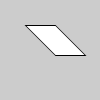Name

### shearX()

Examples```size(100, 100)
translate(width/4, height/4)
shearX(PI/4.0)
rect(0, 0, 30, 30)
```
Description Shears a shape around the x-axis the amount specified by the angle parameter. Angles should be specified in radians (values from 0 to PI*2) or converted to radians with the radians() function. Objects are always sheared around their relative position to the origin and positive numbers shear objects in a clockwise direction. Transformations apply to everything that happens after and subsequent calls to the function accumulates the effect. For example, calling shearX(PI/2) and then shearX(PI/2) is the same as shearX(PI). If shearX() is called within the draw(), the transformation is reset when the loop begins again.

Technically, shearX() multiplies the current transformation matrix by a rotation matrix. This function can be further controlled by the pushMatrix() and popMatrix() functions.
Syntax
```shearX(angle)
```
Parameters
angle float: angle of shear specified in radians
Related popMatrix()
pushMatrix()
shearY()
scale()
translate()